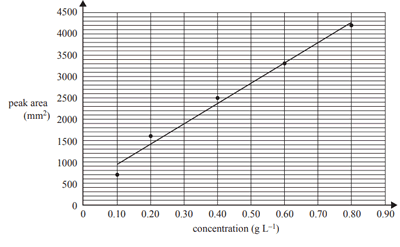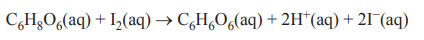Volumetric analysis (2019 VCE) 1)High-performance liquid chromatography (HPLC) was used to determine the sucrose concentration in a sample taken from a can of soft drink. Standard solutions were made up using pure sucrose and deionised water. A 1 mL sample of each standard solution was injected into the HPLC column and its peak area was recorded and used to construct a calibration line of the concentration of sucrose against peak area are shown in the graph below.A 5.0 mL sample of the soft drink was diluted to 100 mL in a volumetric flask. A 10.0 mL aliquot of this solution was transferred to a 250 mL volumetric flask and filled up to the calibration mark using deionised water. A sample of this solution was injected into the HPLC column. The peak area of the sample solution at the same retention time and under the same conditions as those used to determine the calibration line was found to be 1900 mm2. i. Determine the sucrose content of the sample tested in the HPLC, in grams per litre. Solution ii. Calculate the percentage mass/volume (% m/v) of sucrose in the 5.0 mL sample of soft drink Solution iiiThe can used to obtain the sample contained 330 mL of soft drink. Assuming that the only sugar in the soft drink is sucrose, calculate the mass of sucrose in the can of soft drink. Solutionb) Based on the results obtained, is the experimental method valid? Give your reasoning. Solution2) The concentration of vitamin C in a filtered sample of grapefruit juice was determined by titrating the juice with 9.367 × 10-4 M iodine, I2, solution using starch solution as an indicator. The molar mass of vitamin C is 176.0 g mol-1. The reaction can be represented by the following equation.The following method was used: 1. Weigh a clean 250 mL conical flask. 2. Use a 10 mL measuring cylinder to measure 5 mL of grapefruit juice into the conical flask and reweigh it. 3. Add 20 mL of deionised water to the conical flask. 4. Add a drop of starch solution to the conical flask. 5. Titrate the diluted grapefruit juice against the I2 solution a) Which one of the following errors would result in an underestimation of the concentration of vitamin C in grapefruit juice? A. 19 mL of deionised water was added to the conical flask. B. The concentration of the I2 solution was actually 9.178 × 10-4 M. C. The initial volume of the I2 solution in the burette was 1.50 mL, but it was read as 2.50 mL. D. The balance was faulty and the measured mass of grapefruit juice was lower than the actual mass. Solution b) If the measured mass of grapefruit juice was 4.90 g and the titre was 21.50 mL, what was the measured percentage mass/mass (% m/m) concentration of vitamin C in the grapefruit juice? A. 0.00987 B. 0.0723 C. 0.354 D. 3.36 Solution